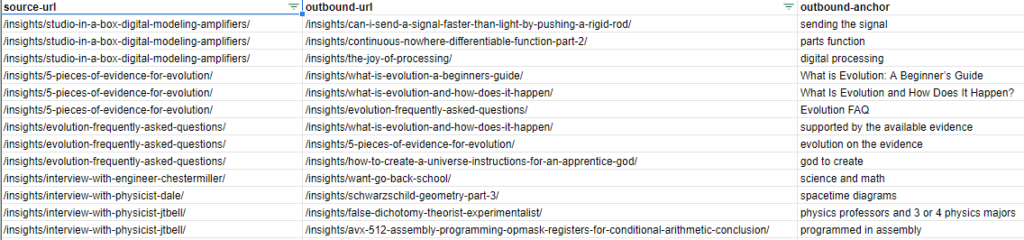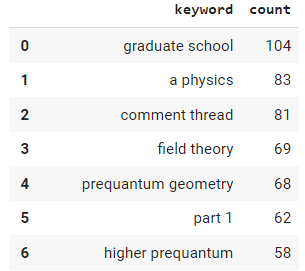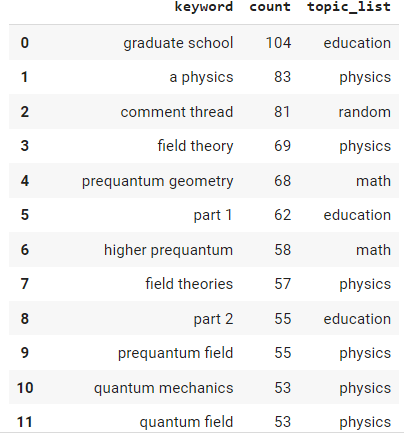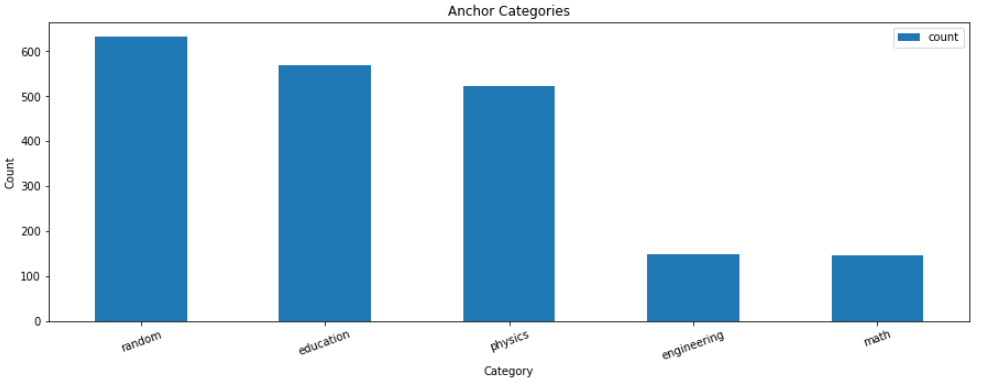Common Topics: anchor, data, text, dataframe, png

In this Python SEO tutorial, I’ll show you a programmatic method to start analyzing your internal anchor text for topical relevance. Internal anchor text remains one of the most powerful topical endorsements you can provide. Anchor texts are explicit contextual signals Google can use to help understand and calculate the linked page’s topical authority. Let’s find out just how topically dense the signals in your anchor text are.

Like most of my SEO tutorials, I will give you a framework and starting point. There are countless approaches, extensions, and further drill-downs for complete analysis. The steps will be as follows:

1. Export an ahrefs internal links report (you could also modify using another platform export like DeepCrawl or even ScreamingFrog)
2. Clean the data
3. N-gram the anchor texts
4. Sort by n-gram frequency
5. Use Huggingface machine learning to classify
6. Graph the results

## Requirements and Assumptions

• Python 3 is installed and basic Python syntax understood
• Very Basic NLP understanding

## Import and Install Python Modules

• nltk: NLP module handles the n-graming and word type tagging
• pandas: for storing and displaying the results
• string: for filtering out punctuation from the anchor text
• collections: for counting n-gram frequency
• transformers: machine learning algorithm for anchor text classification

Let’s first install the Huggingface transformers module we’ll use for the classification step. If you’re using Google Colab remember to put an exclamation point at the beginning.

`pip3 install transformers`

Now we’ll import all the modules I listed above.

```import pandas as pd
import nltk
from nltk.util import ngrams
from nltk.corpus import stopwords
import string
from collections import Counter
from transformers import pipeline```

Next, we need to import the ahrefs internal link report CSV into a dataframe. The CSV in Sheets should look like this format. We will only be using the “outbound-anchor” column.```df = pd.read_csv("ngrams.csv")
df```

## Create N-gram Functions

This next snippet of code is the function to n-gram the anchor text. We take in all the anchors as a string, provide the number of grams we want (1 or 2 is best) and then send them to the NLTK module for processing and what is returned is the list of n-grams.

```def extract_ngrams(data, num):
n_grams = ngrams(nltk.word_tokenize(data), num)
gram_list = [ ' '.join(grams) for grams in n_grams]
return gram_list```

Below we take all the anchors texts in the dataframe, put them into a list, and convert them all to string so we don’t mix data types when we n-gram. Then we join all the anchor texts into one large string and send it to the function we created above to be n-gramed. After the result is returned we do a little data clean-up. We lowercase all the n-grams and filter out punctuation and stop words.

```anchors = [str(x) for x in df['outbound-anchor'].tolist()]
data = ''
data = ' '.join(anchors)
keywords = extract_ngrams(data, 2)
keywords = [x.lower() for x in keywords if x not in string.punctuation]
keywords = [x for x in keywords if ":" not in x]
stop_words = set(stopwords.words('english'))
keywords = [x for x in keywords if not x in stop_words]```

Once we have our cleaned n-gram list we can easily calculate the frequency using the Counter() function in the Collections module. The number in the function can be used to limit how many are calculated. Then we create a dataframe containing those keywords and counts. It should look similar to the dataframe below (but much longer). It’s important to remember that these aren’t always full anchor texts. They are slices intended to classify to determine how topically dense they are, which we are doing next.## Sort N-gram frequency

```keywords = list(Counter(keywords).most_common(100))

df2 = pd.DataFrame(keywords,columns=["keyword","count"])
df2```

Now that we have our bi-gram’d anchor texts we can attempt to classify via a curated topics list that you can create yourself. My site is physics-based so I decided on 6 categories. In order to catch poor anchor text signals, I also include one called “random” which the model often uses when something doesn’t at all fit other categories. The model we’re using is distilbart-mnli-12-9 and is from Huggingface. It’s a zero-shot-classification model meaning that the dataset the model was trained on won’t be specific to our data. I have a full tutorial on the distilbart model here.

## Categorize N-gram with machine learning

```topics =['education','physics','astronomy','math','engineering','random']
classifier = pipeline("zero-shot-classification",
model="valhalla/distilbart-mnli-12-9")```

Once we have our categories set and the model loaded (often over 1gig) we can create the empty lists that will hold the results of each anchor text n-gram processed by the classification model. The loop iterates through the anchor text column, sending the anchor and topic categories to the model. The results are in JSON form so we can easily parse to find the top-scored topic since it evaluates each. Then we simply add the top score and topic to the empty lists we created earlier, rinse and repeat.

```topic_list = []
topic_list_score = []

### iterate through crawl dataframe
for index, row in df2.iterrows():

#### parent topic generation
sequence_to_classify = row.lower()
result = classifier(sequence_to_classify, topics)

### parse results, select top scoring result
topic = result["labels"]
topic_score = str(round(result["scores"],3))

### add result to topic list
topic_list.append(topic)
topic_list_score.append(topic_score)```

Now we simply add the new topics per anchor text n-gram to the dataframe.```df2["topic_list"] = topic_list
df2```

## Graph categories

If you have a small number of anchor texts you may just be able to browse through the rows and get a feel for what is optimal and what isn’t. In the case where you have a large site and could use a visual, we’ll take this extra step and graph our categorical buckets.

We first need to make sure the data is typeset correctly before graphing, then we sum the counts of each topic group in the dataframe and send the data to the bar() plot function.

```df2["count"] = df2["count"].astype(float)
df2["topic_list"] = df2["topic_list"].astype(str)

df2 = df2.groupby(['topic_list'])['count'].sum().to_frame().sort_values('count', ascending=False).reset_index()
df2.plot.bar(x='topic_list', y='count',figsize=(15,5), title="Anchor Categories", xlabel="Category", ylabel="Count", rot=20)```

## Output and ConclusionIt doesn’t take a data scientist to see that I’ve got a problem with my anchor text topical relevance. There are a lot of n-grams that are classified under the random category. The next step would be to examine this bucket further and see where I can improve where these n-grams are actually in anchor text. Anchor text should not be taken for granted and be a strong player in your SEO strategy. Every anchor text should be carefully created with maximum topical relevance signals in mind.

Now you have the start of a framework to begin understanding what topical signals and the strength of those signals are within your anchor text. Remember to try and make my code even more efficient and extend it in ways I never thought of!  Now get out there and try it out! Follow me on Twitter and let me know your applications and ideas!